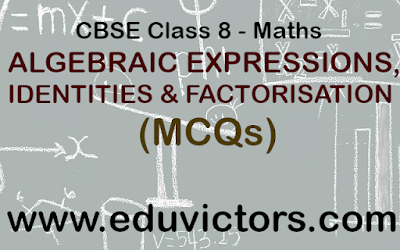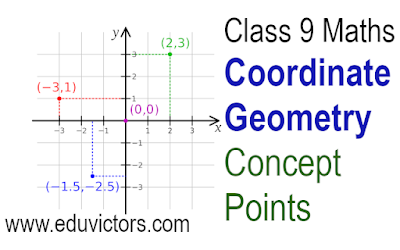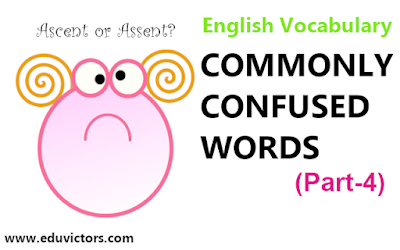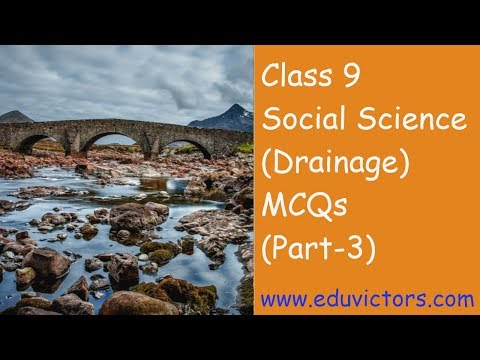## ALGEBRAIC EXPRESSIONS, IDENTITIES AND FACTORISATION

(MCQs)

Q1: Which is the like term as 24a²bc?
(a) 13 × 8a × 2b × c × a
(b) 8 × 3 × a × b × c
(c) 3 × 8 × a × b × c × c
(d) 3 × 8 × a × b × b × c## Coordinate Geometry - Concept PointsClass 9 Maths

1. The system used for describing the position of a point in a plane is called Cartesian system.

2. To locate the position of a point in a plane, we require two perpendicular lines.

3. The plane is called the Cartesian or coordinate plane and the lines are called the coordinate axes.## Commonly Confused Words

(Part-4)
English Vocabulary

English is full of phrases or words, which sound the same or comparable yet have distinctive meanings and are sometimes confusing too. Here is the third list of commonly confused words.

Bad means poor quality is used as an adjective after verbs like am, feel, is,## Laws of Set Operations Class 11 Maths - Sets

Sets under the operations of union, intersection and complement satisfy various laws. These laws collectively called Algebra of sets.

Here follows few important Laws of Set Operations

1. Idempotent Laws:
(a) A ∪ A = A
(b) A ∩ A = A## Class 10 English - Cloze Test (Part 2)

Fill in the Blanks
(New Pattern 2019-2020)

A Cloze Test or Cloze Reading Test is an exercise where the candidates are provided with a passage which has certain words missing from it. It follows logically that to ace such an assessment, you must have a strong command over English Language,  Grammar and Good Vocabulary. More importantly, it is important to understand the flow and context of the passage. Keep reading to know the tips that will help you to solve questions based on Cloze Test.

Directions : In Question Nos. 1 to 10, in the following passage some of the words have been left out. Read the passage carefully and choose the correct answer to each question out of the four alternatives and fill in the blanks.## Class 11  Maths Chapter Sets - NCERT Exercise 1.4 (Q 6 - Q 8)

NCERT Solutions

Q6: If A = { 3, 5, 7, 9, 11 }, B = {7, 9, 11, 13}, C = {11, 13, 15} and D = {15, 17}; find
(i) A ∩ B
(ii) B ∩ C
(iii) A ∩ C ∩ D
(iv) A ∩ C
(v) B ∩ D
(vi) A ∩ (B ∪ C)
(vii) A ∩ D
(viii) A ∩ (B ∪ D)
(ix) ( A ∩ B ) ∩ ( B ∪ C )
(x) ( A ∪ D) ∩ ( B ∪ C)

(i) A ∩ B  = {7,9,11}

(ii) B ∩ C = {11, 13}

(iii) A ∩ C ∩ D = {A ∩ C} ∩ D  = {11} ∩ {15, 17} = φ

(iv) A ∩ C = {11}

(v) B ∩ D = {7, 9, 11, 13}  ∩ {15, 17} = φ

(vi) A ∩ (B ∪ C)
= {3, 5, 7, 9, 11}  ∩ ({7, 9, 11, 13} ∪ {11, 13, 15})
= {3, 5, 7, 9, 11}  ∩ {7, 9, 11, 13, 15}
= {7, 9, 11}

(vii) A ∩ D = φ

(viii) A ∩ (B ∪ D)
= {3, 5, 7, 9, 11}  ∩ ({7, 9, 11, 13} ∪ {15, 17})
= {3, 5, 7, 9, 11}  ∩ {7, 9, 11, 13, 15, 17}
= {7, 9, 11}## Drainage (MCQs)

Class 9 - Geography## Environmental Institutes

General Knowledge
NTSE/CTET/Competitions

Among the enormous number of foundations and institutes that manage ecological assurance and environmental  protection, a few of known institutes and organizations  (some are government-funded) are listed underneath:

1. Arid Zone Research Institute—Jodhpur

2. Central Pollution Control Board—Delhi

3. Central Museum Authority—New Delhi

4. Rehabilitation Institute of Social Forestry and Ecology— Allahabad/Pryagraj

5. G. B, Pant Himalayan Environment and Development Institute— Almora

6. Himalayan Forest Research Centres - Shimla## CBSE Class 11 Maths Sets NCERT Exercise 1.4 Answers

(Q1 - Q5)

Q1: Find the union of each of the following pairs of sets:
(i) X = {1,3,5} Y = {1,2,3}

(ii) A = {a,e,i,o,u}, B = {a,b,c}

(iii) A = {x : x is a natural number and multiple of 3}
B = {x : x is a natural number less than 6}

(iv) A = {x : x is a natural number and 1 < x ≤ 6 }
B = {x : x is a natural number and 6 < x < 10 }

(v) A = {1, 2, 3}, B = φ

(i) X = {1,3,5} Y = {1,2,3}
X ∪ Y = {1,2,3,5}## Sectors of The Indian Economy (Q & A) Part-2

CBSE Class 10 - Economics